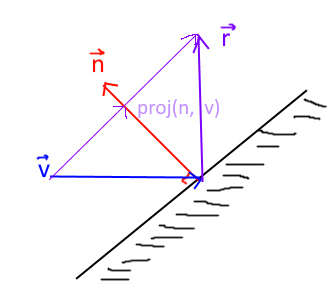# What is wrong with my laser script?

I was playing around with ray casting and I found some odd behavior. I don’t understand. This script was never meant for a game but just for practice and I expect it to bounce off of things and launch but it acts weird. Script is local in startcharscripts
I don’t know much about vector math and stuff but I would love to learn.
Script Below:

``````
local plr = game.Players.LocalPlayer

local mouse = plr:GetMouse()

local maxBounces = 10

local laserParts = workspace:WaitForChild("LaserStuff")

function createPoint(pos)
local part = Instance.new("Part")
part.CanCollide = false
part.CanTouch = false
part.Transparency = 1
part.Locked = true
part.Name = "LaserBouncePoint"
part.Anchored = true
part.Parent = laserParts
local attachment = Instance.new("Attachment")
attachment.Parent = part
return part,attachment
end

function fireLaser()

laserParts:ClearAllChildren()

local params = RaycastParams.new()

local lastResult

local currentVector = headVector * 100

local lastHitPos = nil

local bounces = 0

repeat
local ray = workspace:Raycast(lastPart.Position, currentVector, params)
lastResult = ray
print(ray)
if ray then
currentVector = ray.Normal + (ray.Normal - currentVector) -- should be normal of reflection
currentVector *=100
print(currentVector)
local newPart, attach = createPoint()
local newPart2, attach2 = createPoint() -- Create the beam
if lastHitPos == nil then
else
newPart.Position = lastHitPos
end

lastHitPos = ray.Position

newPart2.Position = ray.Position

local newBeam = Instance.new("Beam")

newBeam.Parent = laserParts

newBeam.Attachment0 = attach

newBeam.Attachment1 = attach2

bounces +=1
end
until lastResult == nil or bounces >= maxBounces
print("done casting")
end

mouse.Button1Down:Connect(function()
fireLaser()
end)
``````

This right here doesn’t look like a reflection formula. It looks close, but isn’t quite correct.
The formula for bouncing a vector off of a surface using it’s normal is

``````reflected = direction - 2 * direction:Dot(normal) * normal -- for vectors facing outwards from the surface

reflected = 2 * direction:Dot(normal) * normal - direction -- for vectors facing inwards towards the surface
``````

Normal should ideally be a unit vector, which I believe ray casts already give a unit vector normal.

The reflected vector would be the same length as the input vector so you wouldn’t need to scale it unless you had specific behavior in mind to make it respond to reflections.The basic idea behind a reflection and why this formula works is that you want to project the
vector onto the normal at a right angle using the properties of a right triangle. You can then get the vector pointing from the input vector to the projected vector, the light purple arrow, and add it to the input vector twice to get a perfectly mirrored reflection vector.

This works because the dot product used in the projection has a cosine in it that describes the angle between the normal and the input vector. You can use this to find the right ratio between the surface normal and the vector that allows you to find the length to make the normal to form a right triangle with the vector.

``````projection = vector:Dot(normal) * normal -- outwards facing vector, though technically works with inwards facing ones too. the projection will just be multiplied by -1 instead
``````

This is only one interpretation of the math though, and there are many different correct ways to interpret it.

3 Likes

Thanks for the help, I am going to try out the changes. I was using the angle of reflection script I used in scratch by finding the angle of incidence and adding it to the surface angle but I guess it’s different.